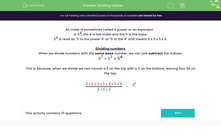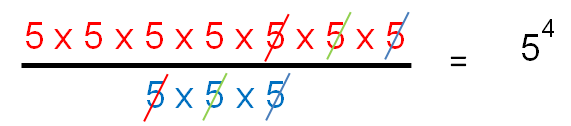# Divide Indices

In this worksheet, students will divide numbers expressed using indices.Key stage:  KS 3

Curriculum topic:   Number

Curriculum subtopic:   Understand Integer Powers/Real Roots

Difficulty level:#### Worksheet Overview

An index is sometimes called a power or an exponent.

In 54, the 4 is the index and the 5 is the base.

54 is read as '5 to the power 4' and means 5 x 5 x 5 x 5.

Dividing numbers

When we divide numbers with the same base number, we can just subtract the indices.

57 ÷ 53 = 54

This is because, when we divide, we can cancel a 5 on the top with a 5 on the bottom, leaving four 5s on the top.Does that make sense?

Let's test it out in the questions.

### What is EdPlace?

We're your National Curriculum aligned online education content provider helping each child succeed in English, maths and science from year 1 to GCSE. With an EdPlace account you’ll be able to track and measure progress, helping each child achieve their best. We build confidence and attainment by personalising each child’s learning at a level that suits them.

Get started